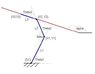# Serial Link chain with constrained geometry

• nucloxylon
In summary, the conversation discusses a three link revolute manipulator at the origin with known link lengths and a joint angle constraint. The goal is to determine the joint angles and positions of the manipulator, but the system is over-constrained and a unique solution cannot be found.

#### nucloxylon

I have a three link revolute manipulator at the origin. I know all the link lengths. The joint angle for the third link is coupled to the second such that Theta3 = k*Theta2. I want to determine the joint angles (thetas) of the manipulator given that the third link should lie on a line an angle Alpha from the x - axis at a known position.

I believe the solution should be unique but I can't seem to wrap my hands around an equation that proves it.

To summarize:
Knowns:
Angle of line: Alpha = Theta1 + Theta2 + Theta3 = Theta1 + Theta2(1+k)
And it's position
Coupling function: Theta3 = Theta2 * k

Unknowns
Theta1, Theta2, Theta3
Joint positions, X1,Y1, X2, Y2, X3, Y3

I tried assuming I knew one of the joint end positions, i.e X2, Y2, because my intuition tells me there's got to be only one solution. Then maybe I could find another set of equations and somehow cut it out. Here's my work so far:

By law of cosines:
X2^2 + Y2^2 = L1^2 + L2^2 - 2L1L2cos(beta)
where beta is 180 - Theta2 (see diagram)
beta = acos((L1^2 + L2^2 - (X2^2 + Y2^2)) / (2L1L2))
Theta2 = 180 - beta
Theta3 = (180 - beta) * k
Theta1 = alpha - Theta2 - Theta3

But now I'm stuck because I can't come up with another good set of equations to remove X2 and Y2 since I don't actually know them. Any insight or advice would be great.

Thanks!

#### Attachments

•manipulator.JPG
11.5 KB · Views: 451
Last edited:
nucloxylon said:
I believe the solution should be unique but I can't seem to wrap my hands around an equation that proves it.
The link lengths are known and fixed. L1, L2 and L3.
Given the specified point x3, y3 and alpha, we can compute the position of x2, y2.

There are then two circles, one has radius L1, with centre at the origin.
The other circle has radius L2, with centre at x2, y2.
The intersection points of the two circles, (if they do intersect), give the two possible solutions for x1, y1.

Unfortunately the theta3 = k * theta2 constraint has not been satisfied.
It is clear that movement of link3 along the alpha line will force the value of k to be changed.
So the system is over-constrained.
Which explains why the equations cannot be solved.

## What is a Serial Link chain with constrained geometry?

A Serial Link chain with constrained geometry is a type of mechanical linkage system that consists of multiple links connected in a specific sequence and constrained by geometric constraints. This type of chain is often used in robotics and other mechanical systems to achieve precise movements and control.

## What are the benefits of using a Serial Link chain with constrained geometry?

There are several benefits to using a Serial Link chain with constrained geometry. Firstly, it allows for precise and controlled movements, making it ideal for tasks that require high accuracy. Additionally, it can be designed with a compact size, making it suitable for use in small spaces. Lastly, it can be easily programmed and controlled, making it versatile for various applications.

## What are the different types of geometric constraints used in a Serial Link chain?

There are several types of geometric constraints used in a Serial Link chain, including revolute, prismatic, cylindrical, and spherical constraints. Revolute constraints allow for rotational movement between two links, while prismatic constraints allow for linear motion. Cylindrical and spherical constraints are used to restrict movement in multiple directions.

## What factors should be considered when designing a Serial Link chain with constrained geometry?

When designing a Serial Link chain with constrained geometry, factors such as the desired movement, load capacity, precision, and size constraints should be carefully considered. The type and location of the constraints, as well as the number and lengths of the links, should also be taken into account to achieve the desired performance.

## What are some real-world applications of Serial Link chains with constrained geometry?

Serial Link chains with constrained geometry have a wide range of applications, including robotics, manufacturing, medical devices, and aerospace engineering. They are commonly used in robotic arms, automated assembly lines, surgical robots, and space exploration vehicles. They can also be found in everyday items such as car engines and printers.